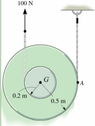# Solving for 'a' with Torque, Force, and Mass Moment of Inertia

• haven

#### haven

Homework Statement
The 7.80 kg spool has a radius of gyration of 0.320 m and rolls without the ropes slipping. The ropes have negligible mass.

There is also a force pulling the spool upward at 100N and the outer radius is 0.5 and the inner radius is 0.2-shown in picture
Let up be the positive y-direction and CCW be the positive rotational direction.
Assume 3 SF for all givens.
Relevant Equations
Torque = I*a
Io=m*ro^2
What I did was plug in the outer radius time the force into the torque and then the mass moment of inertia is equal to m*ro^2 so then I plugged in the mass times the radius of gyration squared into I and solved for a but this is not right.

#### Attachments

•Screen Shot 2022-11-27 at 6.14.19 PM.png
37.8 KB · Views: 30
Is there a figure that comes with this? If so, please post it using the "Attach files" link, lower left.

Is there a figure that comes with this? If so, please post it using the "Attach files" link, lower left.
yes I just attached it^^

Thank you for posting the figure. Draw a free body diagram (FBD) of the wheel.

Note that the center of the wheel has a linear acceleration and there is also angular acceleration about the center of the wheel. So you need to write two equations, one for the linear acceleration and one for the angular acceleration. Don't forget the tension in the rope that is attached to the ceiling; it too exerts a force and a torque on the wheel. Gravity also affects the motion and your solution does not seem to have taken it into account.